# Winter 2023 Final Exam

Instructor(s): Suraj Rampure

This exam was administered in-person. The exam was closed-notes, except students were allowed to bring 2 two-sided cheat sheets. No calculators were allowed. Students had 180 minutes to take this exam.

The DataFrame sat contains one row for most combinations of "Year" and "State", where "Year"ranges between 2005 and 2015 and "State" is one of the 50 states (not including the District of Columbia).

The other columns are as follows:

• "# Students" contains the number of students who took the SAT in that state in that year.

• "Math" contains the mean math section score among all students who took the SAT in that state in that year. This ranges from 200 to 800.

• "Verbal" contains the mean verbal section score among all students who took the SAT in that state in that year. This ranges from 200 to 800. (This is now known as the “Critical Reading” section.)

The first few rows of sat are shown below (though sat has many more rows than are pictured here).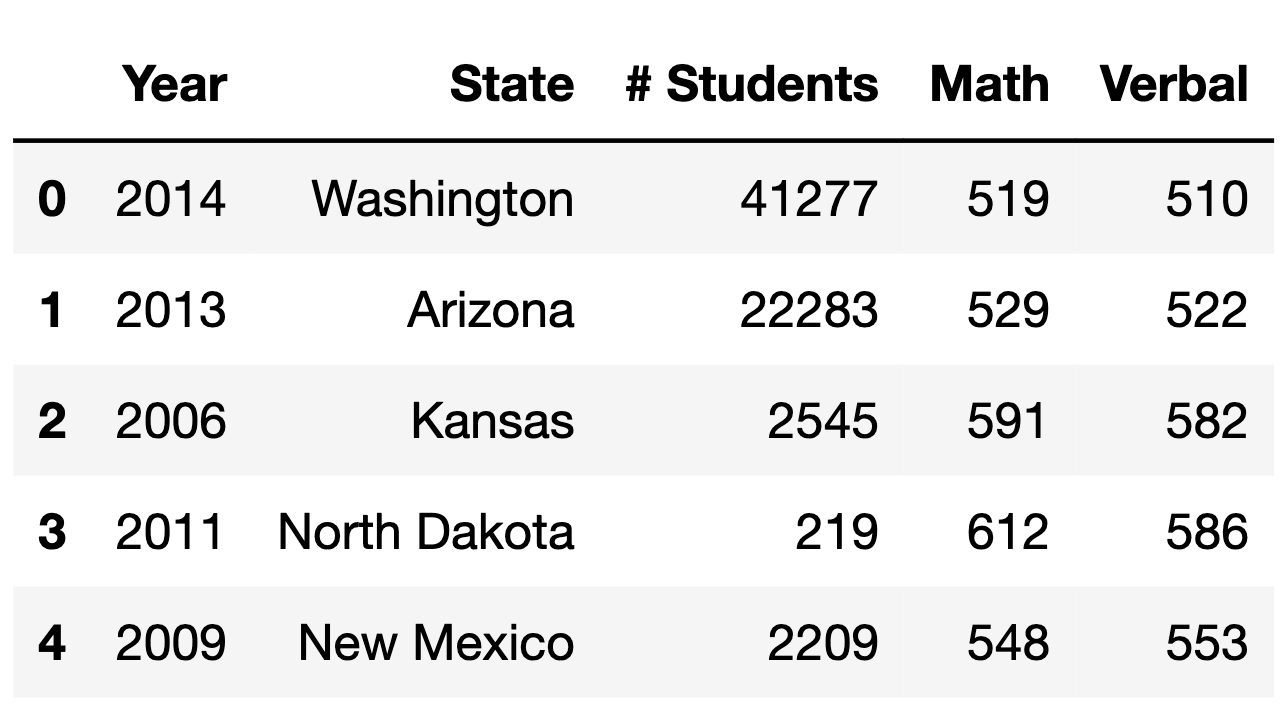For instance, the first row of sat tells us that 41227 students took the SAT in Washington in 2014, and among those students, the mean math score was 519 and the mean verbal score was 510.

Assume:

• sat does not contain any duplicate rows — that is, there is only one row for every unique combination of "Year" and "State" that is in sat.

• sat does not contain any null values.

• We have already run all of the necessary imports.

## Problem 1

### Problem 1.1

Which of the following expressions evaluate to the name of the state, as a string, with the highest mean math section score in 2007? Select all that apply.

Note: Assume that the highest mean math section score in 2007 was unique to only one state.

Option 1:

(sat.loc[(sat["Math"] == sat["Math"].max()) &
(sat["Year"] == 2007), "State"]
.iloc)

Option 2:

sat.loc[sat["Year"] == 2007].set_index("State")["Math"].idxmax()

Option 3:

sat.groupby("Year")["State"].max().loc

Option 4:

(sat.loc[sat["Math"] == sat.loc[sat["Year"] == 2007, "Math"].max()]
.iloc
.loc["State"])

Option 5:

(sat.groupby("Year").apply(
lambda sat: sat[sat["Math"] == sat["Math"].max()]
).reset_index(drop=True)
.groupby("Year")["State"].max()
.loc)

Option 6:

sat.loc[sat['Year'] == 2007].loc[sat['Math'] == sat['Math'].max()]
• Option 1

• Option 2

• Option 3

• Option 4

• Option 5

• Option 6

• None of the above

Answer: Option 2 and Option 5

### Problem 1.2

In the box, write a one-line expression that evaluates to a DataFrame that is equivalent to the following relation:

\Pi_{\text{Year, State, Verbal}} \left(\sigma_{\text{Year } \geq \: 2014 \text{ and Math } \leq \: 600} \left( \text{sat} \right) \right)

Answer: sat.loc[(sat['Year'] >= 2014) & (sat['Math'] <= 600), ['Year', 'State', 'Verbal']]

### Problem 1.3

The following two lines define two DataFrames, val1 and val2.

val1 = sat.groupby(["Year", "State"]).max().reset_index()
val2 = sat.groupby(["Year", "State", "# Students"]).min().reset_index()

Are val1 and val2 identical? That is, do they contain the same rows and columns, all in the same order?

• Yes

• No

### Problem 1.4

The data description stated that there is one row in sat for most combinations of "Year" (between 2005 and 2015, inclusive) and "State". This means that for most states, there are 11 rows in sat — one for each year between 2005 and 2015, inclusive.

It turns out that there are 11 rows in sat for all 50 states, except for one state. Fill in the blanks below so that missing_years evaluates to an array, sorted in any order, containing the years for which that one state does not appear in sat.

state_only = sat.groupby("State").filter(___(a)___)

merged = sat["Year"].value_counts().to_frame().merge(
state_only, ___(b)___
)

missing_years = ___(c)___.to_numpy()

What goes in blank (a)?

What goes in blank (b)?

What goes in blank (c)?

Answer: a: lambda df: df.shape < 11, b: left_index=True, right_on='Year', how='left' (how='outer' also works), c: merged[merged['# Students'].isna()]['Year']

In the previous subpart, we established that most states have 11 rows in sat — one for each year between 2005 and 2015, inclusive — while there is one state that has fewer than 11 rows, because there are some years for which that state’s SAT information is not known.

Suppose we’re given a version of sat called sat_complete that has all of the same information as sat, but that also has rows for combinations of states and years in which SAT information is not known. While there are no null values in the "Year" or "State" columns of sat_complete, there are null values in the "# Students", "Math", and "Verbal" columns of sat_complete. An example of what sat_complete may look like is given below.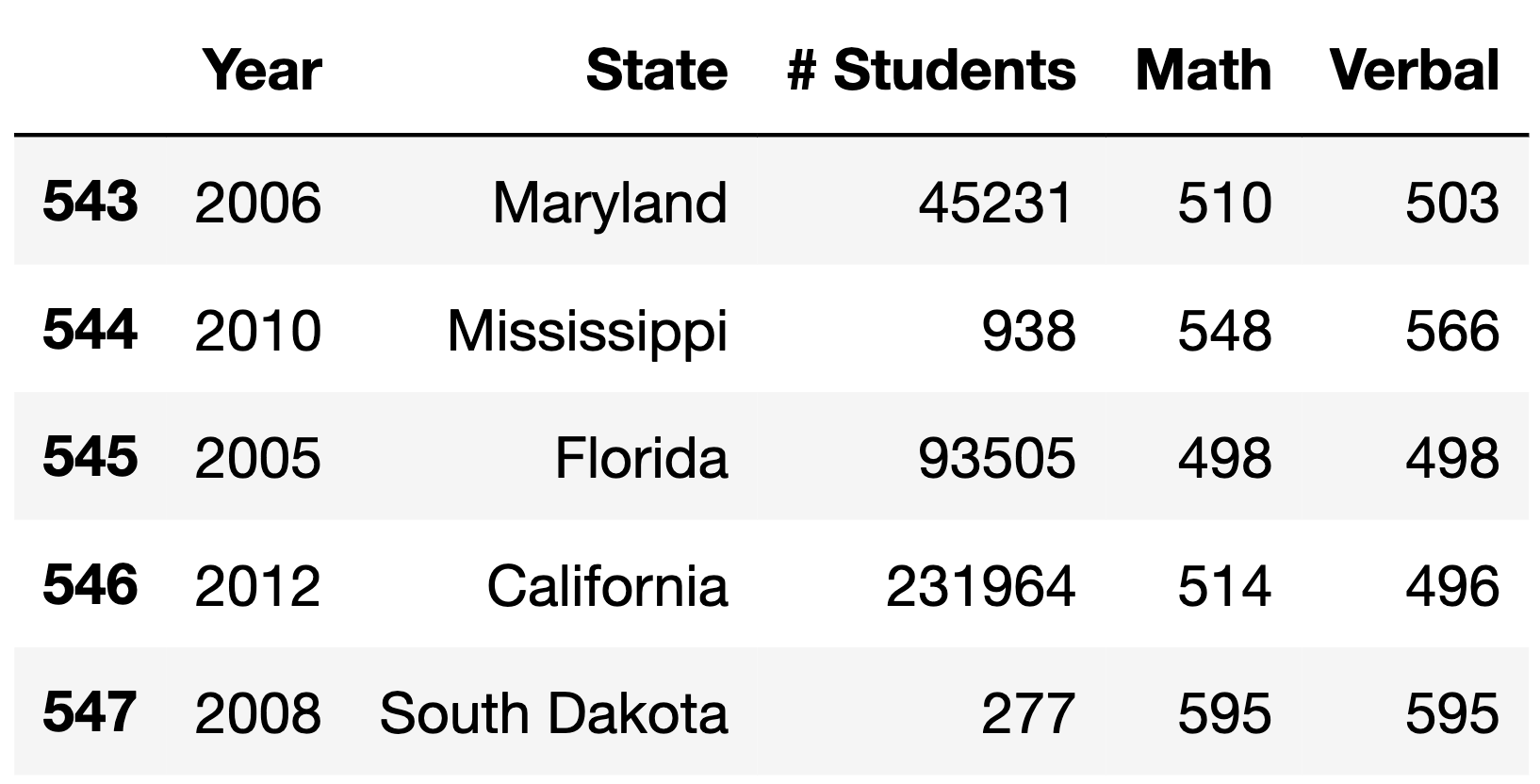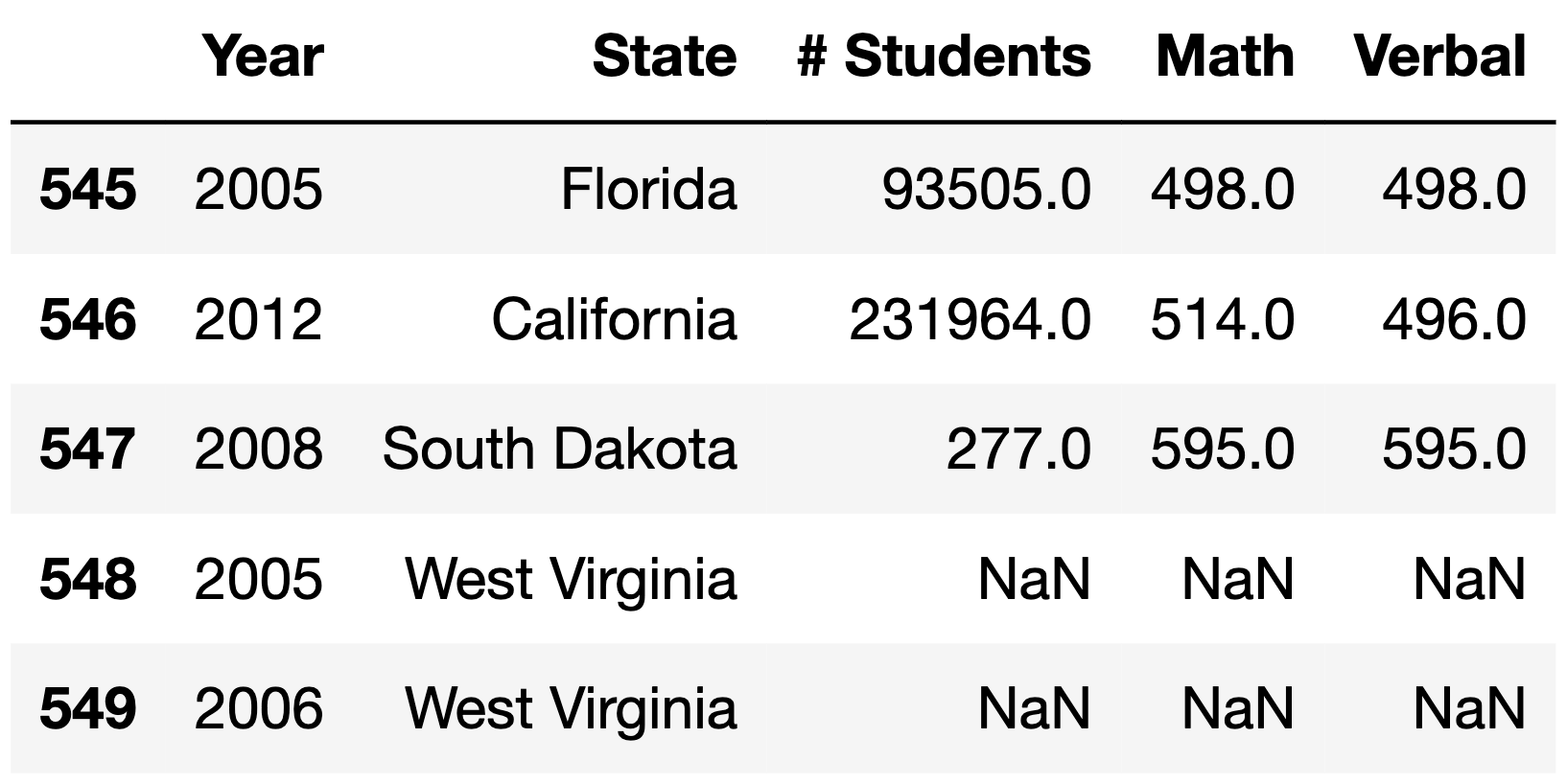Note that in the above example, sat simply wouldn’t have rows for West Virginia in 2005 and 2006, meaning it would have 2 fewer rows than the corresponding sat_complete.

### Problem 1.5

Given just the information in sat_complete — that is, without including any information learned in part (d) — what is the most likely missingness mechanism of the "# Students" column in sat_complete?

• Not missing at random

• Missing at random

• Missing completely at random

### Problem 1.6

Given just the information in sat_complete — that is, without including any information learned in part (d) — what is the most likely missingness mechanism of the "Math" column in sat_complete?

• Not missing at random

• Missing at random

• Missing completely at random

### Problem 1.7

Suppose we perform a permutation test to assess whether the missingness of column Y depends on column X.

Suppose we observe a statistically significant result (that is, the p-value of our test is less than 0.05). True or False: It is still possible for column Y to be not missing at random.

• True

• False

### Problem 1.8

Suppose we do not observe a statistically significant result (that is, the p-value of our test is greater than 0.05). True or False: It is still possible for column Y to be missing at random dependent on column X.

• True

• False

## Problem 2

The following DataFrame contains the mean, median, and standard deviation of the number of students per year who took the SAT in New York and Texas between 2005 and 2015.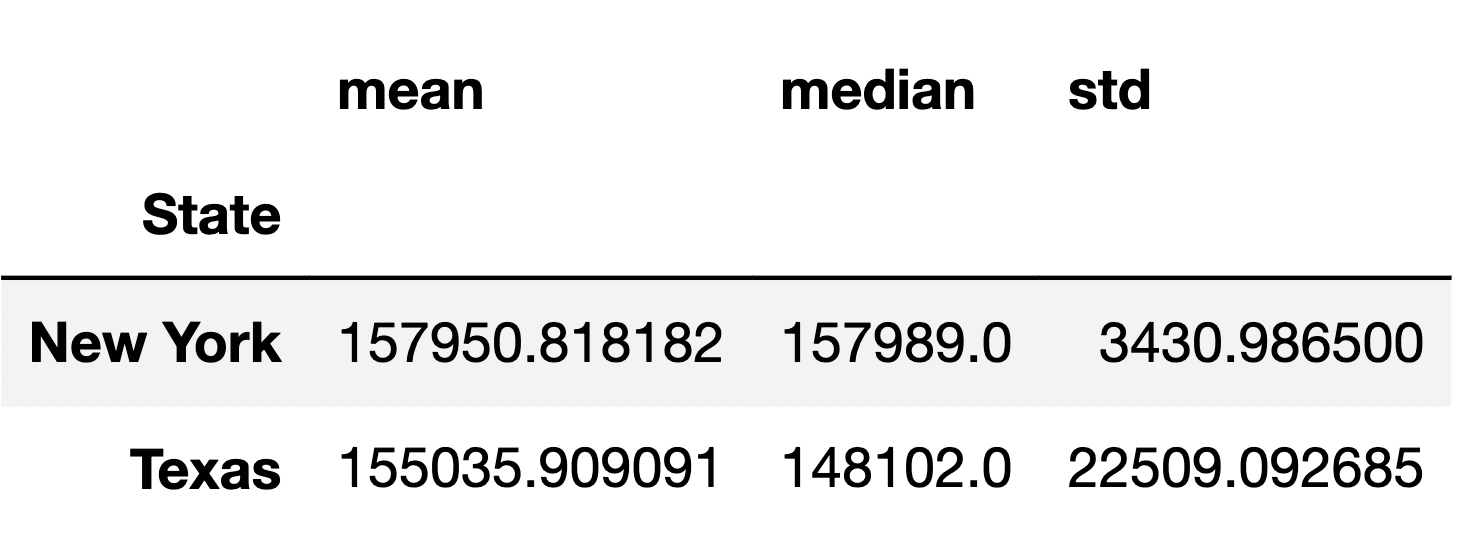### Problem 2.1

Which of the following expressions creates the above DataFrame correctly and in the most efficient possible way (in terms of time and space complexity)?

Note: The only difference between the options is the positioning of "# Students".

Option 1:

(sat.loc[sat["State"].isin(["New York", "Texas"])]
["# Students"].groupby("State").agg(["mean", "median", "std"]))

Option 2:

(sat.loc[sat["State"].isin(["New York", "Texas"])]
.groupby("State")["# Students"].agg(["mean", "median", "std"]))

Option 3:

(sat.loc[sat["State"].isin(["New York", "Texas"])]
.groupby("State").agg(["mean", "median", "std"])["# Students"])
• Option 1

• Option 2

• Option 3

• Multiple options are equally correct and efficient

Suppose we want to run a statistical test to assess whether the distributions of the number of students between 2005 and 2015 in New York and Texas are significantly different.

### Problem 2.2

What type of test is being proposed above?

( ) Hypothesis test ( ) Permutation test

### Problem 2.3

Given the information in the above DataFrame, which test statistic is most likely to yield a significant difference?

• \text{mean number of students in Texas } - \text{ mean number of students in New York}

• \big|\text{mean number of students in Texas } - \text{ mean number of students in New York}\big|

• \big|\text{median number of students in Texas } - \text{ median number of students in New York}\big|

• The Kolmogorov-Smirnov statistic

Now, suppose we’re interested in comparing the verbal score distribution of students who took the SAT in New York in 2015 to the verbal score distribution of all students who took the SAT in 2015.

The DataFrame scores_2015, shown in its entirety below, contains the verbal section score distributions of students in New York in 2015 and for all students in 2015.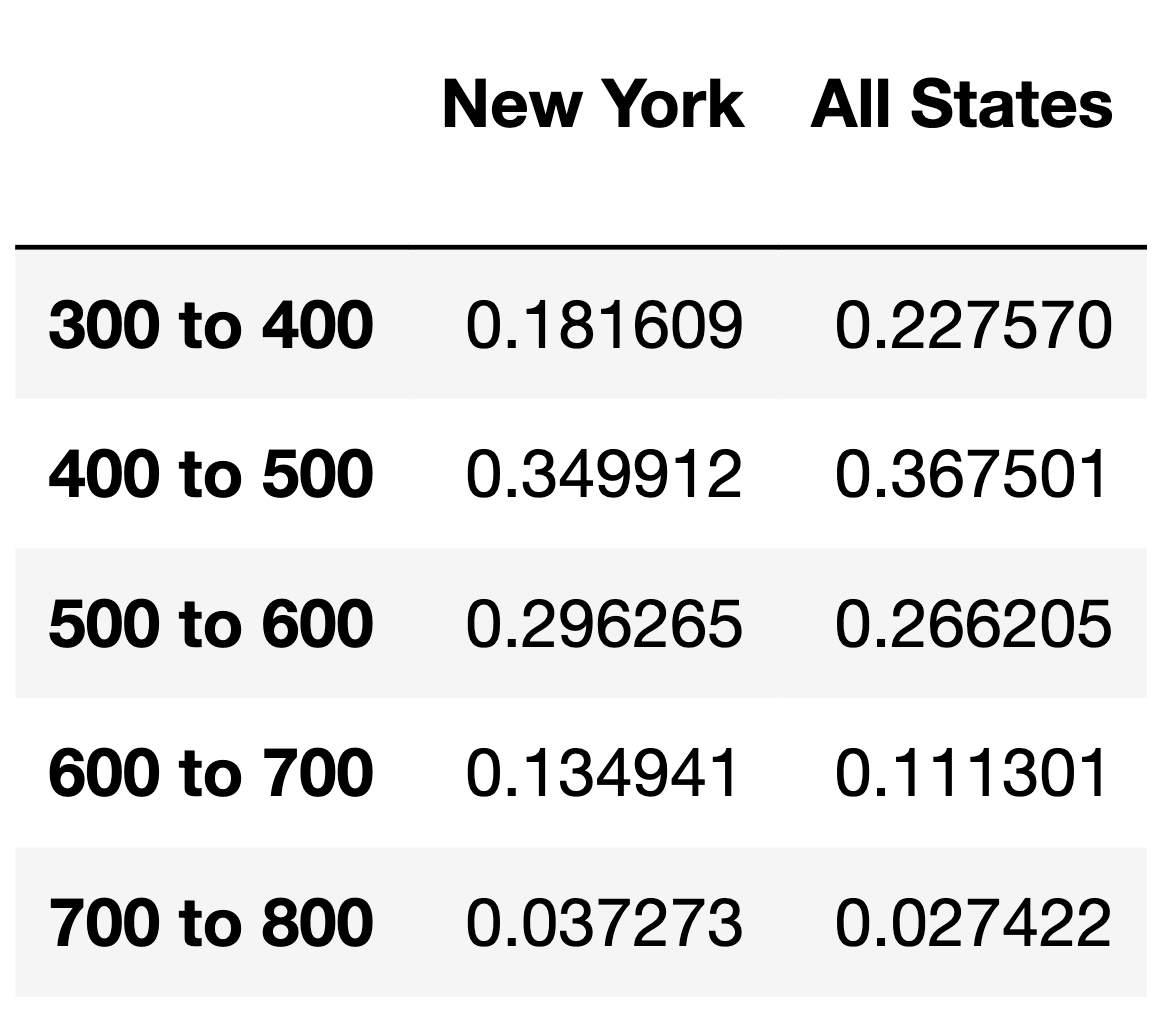### Problem 2.4

What type of test is being proposed above?

( ) Hypothesis test ( ) Permutation test

### Problem 2.5

Suppose \vec{a} = \begin{bmatrix} a_1 & a_2 & ... & a_n \end{bmatrix}^T and \vec{b} = \begin{bmatrix} b_1 & b_2 & ... & b_n \end{bmatrix}^T are both vectors containing proportions that add to 1 (e.g. \vec{a} could be the "New York" column above and \vec{b} could be the "All States" column above). As we’ve seen before, the TVD is defined as follows:

\text{TVD}(\vec{a}, \vec{b}) = \frac{1}{2} \sum_{i = 1}^n \left| a_i - b_i \right|

The TVD is not the only metric that can quantify the distance between two categorical distributions. Here are three other possible distance metrics:

• \text{dis1}(\vec{a}, \vec{b}) = \vec{a} \cdot \vec{b} = a_1b_1 + a_2b_2 + ... + a_nb_n

• \text{dis2}(\vec{a}, \vec{b}) = \frac{\vec{a} \cdot \vec{b}}{|\vec{a} | | \vec{b} |} = \frac{a_1b_1 + a_2b_2 + ... + a_nb_n}{\sqrt{a_1^2 + a_2^2 + ... + a_n^2} \sqrt{b_1^2 + b_2^2 + ... + b_n^2}}

• text{dis3}(\vec{a}, \vec{b}) = 1 - \frac{\vec{a} \cdot \vec{b}}{|\vec{a} | | \vec{b} |}

Of the above three possible distance metrics, only one of them has the same range as the TVD (i.e. the same minimum possible value and the same maximum possible value) and has the property that smaller values correspond to more similar vectors. Which distance metric is it?

• \text{dis1}

• \text{dis2}

• \text{dis3}

## Problem 3

The function state_perm is attempting to implement a test of the null hypothesis that the distributions of mean math section scores between 2005 and 2015 for two states are drawn from the same population distribution.

def state_perm(states):
if len(states) != 2:
raise ValueError(f"Expected 2 elements, got {len(states)}")

def calc_test_stat(df):
return df.groupby("State")["Math"].mean().abs().diff().iloc[-1]

states = sat.loc[sat["State"].isin(states), ["State", "Math"]]

test_stats = []
for _ in range(10000):
states["State"] = np.random.permutation(states["State"])
test_stat = calc_test_stat(states)
test_stats.append(test_stat)

obs = calc_test_stat(states)
return (np.array(test_stats) >= obs).mean()

Suppose we call state_perm(["California", "Washington"]) and see 0.514.

### Problem 3.1

What test statistic is being used in the above call to state_perm?

• \text{mean Washington score } - \text{mean California score}

• \text{mean California score } - \text{mean Washington score}

• \big|\text{mean Washington score } - \text{mean California score} \big|

### Problem 3.2

There is exactly one issue with the implementation of state_perm. In exactly one sentence, identify the issue and state how you would fix it.

Hint: The issue is not with the implementation of the function calc_test_stat.

Answer: Since we are permuting in-place on the states DataFrame, we must calculate the observed test statistic before we permute.

## Problem 4

To prepare for the verbal component of the SAT, Nicole decides to read research papers on data science. While reading these papers, she notices that there are many citations interspersed that refer to other research papers, and she’d like to read the cited papers as well.

In the papers that Nicole is reading, citations are formatted in the verbost numeric style. An excerpt from one such paper is stored in the string s below.

s = '''
In DSC 10 , you learned about babypandas, a strict subset
of pandas . It was designed  to provide programming
beginners  just enough syntax to be able to perform
meaningful tabular data analysis  without getting lost in
100s of details.
'''    

We decide to help Nicole extract citation numbers from papers. Consider the following four extracted lists.

list1 = ['10', '100']
list2 = ['3', '15', '4', '5', '3', '91', '8']
list3 = ['10', '3', '15', '4', '5', '3', '91', '8', '100']
list4 = ['', '', '', '', '', '', '']
list5 = ['1', '0', '3', '1', '5', '4', '5', '3',
'9', '1', '8', '1', '0', '0']

For each expression below, select the list it evaluates to, or select “None of the above.”

### Problem 4.1

re.findall(r'\d+', s)

( ) list1 ( ) list2 ( ) list3 ( ) list4 ( ) list5 ( ) None of the above

### Problem 4.2

re.findall(r'[\d+]', s)

( ) list1 ( ) list2 ( ) list3 ( ) list4 ( ) list5 ( ) None of the above

### Problem 4.3

re.findall(r'$(\d+)$', s)

( ) list1 ( ) list2 ( ) list3 ( ) list4 ( ) list5 ( ) None of the above

### Problem 4.4

re.findall(r'($\d+$)', s)

( ) list1 ( ) list2 ( ) list3 ( ) list4 ( ) list5 ( ) None of the above

## Problem 5

After taking the SAT, Nicole wants to check the College Board’s website to see her score. However, the College Board recently updated their website to use non-standard HTML tags and Nicole’s browser can’t render it correctly. As such, she resorts to making a GET request to the site with her scores on it to get back the source HTML and tries to parse it with BeautifulSoup.

Suppose soup is a BeautifulSoup object instantiated using the following HTML document.

<college>Your score is ready!</college>

<scorelist listtype="percentiles">
<scorerow kind="verbal" subkind="per">
Verbal: <scorenum>84</scorenum>
</scorerow>
<scorerow kind="math" subkind="per">
Math: <scorenum>99</scorenum>
</scorerow>
</scorelist>
And your actual scores are as follows:
<scorelist listtype="scores">
<scorerow kind="verbal">
Verbal: <scorenum>680</scorenum>
</scorerow>
<scorerow kind="math">
Math: <scorenum>800</scorenum>
</scorerow>
</scorelist>
</sat>

### Problem 5.1

Which of the following expressions evaluate to “verbal”}? Select all that apply.

• soup.find("scorerow").get("kind")

• soup.find("sat").get("ready")

• soup.find("scorerow").text.split(":").lower()

• [s.get("kind") for s in soup.find_all("scorerow")][-2]

• soup.find("scorelist", attrs={"listtype":"scores"}).get("kind")

• None of the above

Answer: Option 1, Option 3, Option 4

### Problem 5.2

(6 pts) Consider the following function.

def summer(tree):
if isinstance(tree, list):
total = 0
for subtree in tree:
for s in subtree.find_all("scorenum"):
total += int(s.text)
else:
return sum([int(s.text) for s in tree.find_all("scorenum")])

For each of the following values, fill in the blanks to assign tree such that summer(tree) evaluates to the desired value. The first example has been done for you.

• Desired value: 84
    tree = soup.find(_____)

"scorerow"

• Desired value: 183
    tree = soup.find(__a__)
• Desired value: 1480
    tree = soup.find(__b__)
• Desired value: 899
    tree = soup.find_all(__c__)

Answer: a: "scorelist", b: "scorelist", attrs={"listtype":"scores"}, c: "scorerow", attrs={"kind":"math"}

## Problem 6

Consider the following list of tokens.

py tokens = ["is", "the", "college", "board", "the", "board", "of", "college"] "

### Problem 6.1

Recall, a uniform language model is one in which each unique token has the same chance of being sampled. Suppose we instantiate a uniform language model on tokens. The probability of the sentence “““the college board is” — that is, P(\text{the college board is}) — is of the form \frac{1}{a^b}, where a and b are both positive integers.

What are a and b?

Answer: a = 5, b = 4

### Problem 6.2

Recall, a unigram language model is one in which the chance that a token is sampled is equal to its observed frequency in the list of tokens. Suppose we instantiate a unigram language model on tokens. The probability P(\text{the college board is}) is of the form \frac{1}{c^d}, where c and d are both positive integers.

What are c and d?

Answer: (c, d) = (2, 9) or (8, 3)

For the remainder of this question, consider the following five sentences.

• "of the college board the"
• "the board the board the"
• "board the college board of"
• "the college board of college"
• "board the college board is"

### Problem 6.3

Recall, a bigram language model is an N-gram model with N=2. Suppose we instantiate a bigram language model on tokens. Which of the following sentences of length 5 is the most likely to be sampled?

• Sentence 1

• Sentence 2

• Sentence 3

• Sentence 4

• Sentence 5

For your convenience, we repeat the same five sentences again below.

• "of the college board the"
• "the board the board the"
• "board the college board of"
• "the college board of college"
• "board the college board is"

Suppose we create a TF-IDF matrix, in which there is one row for each sentence and one column for each unique word. The value in row i and column j is the TF-IDF of word j in sentence i. Note that since there are 5 sentences and 5 unique words across all sentences, the TF-IDF matrix has 25 total values.

### Problem 6.4

Is there a column in the TF-IDF matrix in which all values are 0?

• Yes

• No

### Problem 6.5

In which of the following sentences is “college” the word with the highest TF-IDF?

• Sentence 1

• Sentence 2

• Sentence 3

• Sentence 4

• Sentence 5

### Problem 6.6

As an alternative to TF-IDF, Yuxin proposes the DF-ITF, or “document frequency-inverse term frequency”. The DF-ITF of term t in document d is defined below:

\text{df-itf}(t, d) = \frac{\text{\# of documents in which $t$ appears}}{\text{total \# of documents}} \cdot \log \left( \frac{\text{total \# of words in $d$}}{\text{\# of occurrences of $t$ in $d$}} \right)

Fill in the blank: The term t in document d that best summarizes document d is the term with ____.

• the largest DF-ITF in document d

• the smallest DF-ITF in document d

## Problem 7

We decide to build a classifier that takes in a state’s demographic information and predicts whether, in a given year:

• The state’s mean math score was greater than its mean verbal score (1), or

• the state’s mean math score was less than or equal to its mean verbal score (0).

### Problem 7.1

(2 pts) The simplest possible classifier we could build is one that predicts the same label (1 or 0) every time, independent of all other features.

Consider the following statement:

If a > b, then the constant classifier that maximizes training accuracy predicts 1 every time; otherwise, it predicts 0 every time.

For which combination of a and b is the above statement not guaranteed to be true?

Note: Treat as our training set.

Option 1:

a = (sat['Math'] > sat['Verbal']).mean()
b = 0.5

Option 2:

a = (sat['Math'] - sat['Verbal']).mean()
b = 0

Option 3:

a = (sat['Math'] - sat['Verbal'] > 0).mean()
b = 0.5

Option 4:

a = ((sat['Math'] / sat['Verbal']) > 1).mean() - 0.5
b = 0
• Option 1

• Option 2

• Option 3

• Option 4

### Problem 7.2

Suppose we train a classifier, named Classifier 1, and it achieves an accuracy of \frac{5}{9} on our training set.

Typically, root mean squared error (RMSE) is used as a performance metric for regression models, but mathematically, nothing is stopping us from using it as a performance metric for classification models as well.

What is the RMSE of Classifier 1 on our training set? Give your answer as a simplified fraction.

### Problem 7.3

While Classifier 1’s accuracy on our training set is \frac{5}{9}, its accuracy on our test set is \frac{1}{4}. Which of the following scenarios is most likely?

• Classifier 1 overfit to our training set; we need to increase its complexity.

• Classifier 1 overfit to our training set; we need to decrease its complexity.

• Classifier 1 underfit to our training set; we need to increase its complexity.

• Classifier 1 underfit to our training set; we need to decrease its complexity.

For the remainder of this question, suppose we train another classifier, named Classifier 2, again on our training set. Its performance on the training set is described in the confusion matrix below. Note that the columns of the confusion matrix have been separately normalized so that each has a sum of 1.### Problem 7.4

Suppose conf is the DataFrame above. Which of the following evaluates to a Series of length 2 whose only unique value is the number 1?

• conf.sum(axis=0)

• conf.sum(axis=1)

### Problem 7.5

Fill in the blank: the ___ of Classifier 2 is guaranteed to be 0.6.

• precision

• recall

For your convenience, we show the column-normalized confusion matrix from the previous page below. You will need to use the specific numbers in this matrix when answering the following subpart.### Problem 7.6

Suppose a fraction \alpha of the labels in the training set are actually 1 and the remaining 1 - \alpha are actually 0. The accuracy of Classifier 2 is 0.65. What is the value of \alpha?

Hint: If you’re unsure on how to proceed, here are some guiding questions:

• Suppose the number of y-values that are actually 1 is A and that the number of y-values that are actually 0 is B. In terms of A and B, what is the accuracy of Classifier 2? Remember, you’ll need to refer to the numbers in the confusion matrix above.

• What is the relationship between A, B, and \alpha? How does it simplify your calculation for the accuracy in the previous step?

## Problem 8

Let’s continue with the premise from the previous question. That is, we will aim to build a classifier that takes in demographic information about a state from a particular year and predicts whether or not the state’s mean math score is higher than its mean verbal score that year.

In honor of the rotisserie chicken event on UCSD’s campus a few weeks ago, sklearn released a new classifier class called ChickenClassifier.

### Problem 8.1

ChickenClassifiers have many hyperparameters, one of which is height. As we increase the value of height, the model variance of the resulting ChickenClassifier also increases.

First, we consider the training and testing accuracy of a ChickenClassifier trained using various values of height. Consider the plot below.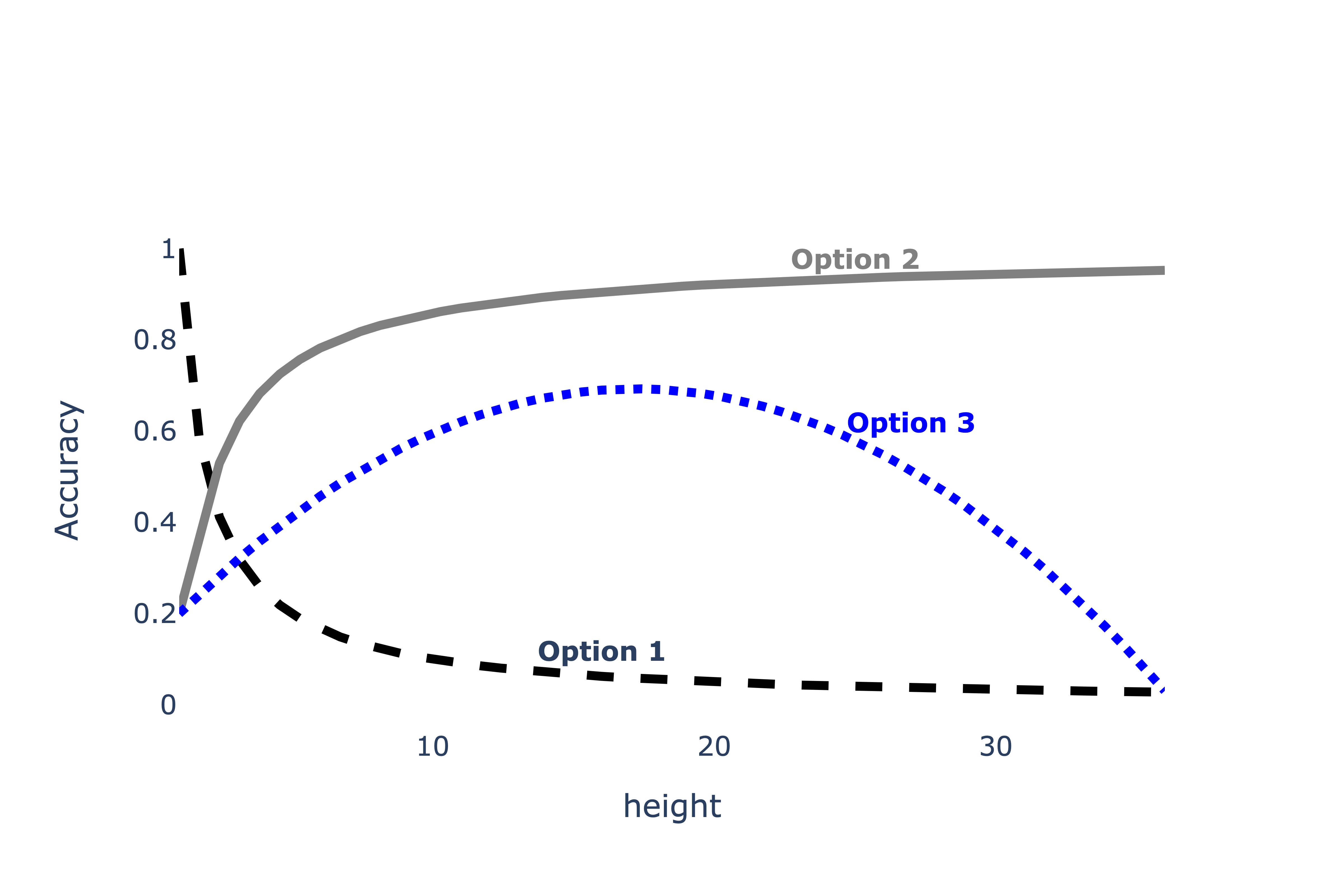Which of the following depicts training accuracy vs. height?

• Option 1

• Option 2

• Option 3

Which of the following depicts testing accuracy vs. height?

• Option 1

• Option 2

• Option 3

### Problem 8.2

ChickenClassifiers have another hyperparameter, color, for which there are four possible values: "yellow", "brown", "red", and "orange". To find the optimal value of color, we perform k-fold cross-validation with k=4. The results are given in the table below.Which value of color has the best average validation accuracy?

• "yellow"

• "brown"

• "red"

• "orange"

True or False: It is possible for a hyperparameter value to have the best average validation accuracy across all folds, but not have the best validation accuracy in any one particular fold.

• True

• False

Answer: "red", True

### Problem 8.3

Now, instead of finding the best height and best color individually, we decide to perform a grid search that uses k-fold cross-validation to find the combination of height and color with the best average validation accuracy.

For the purposes of this question, assume that: - We are performing k-fold cross validation.

• Our training set contains n rows, where n is greater than 5 and is a multiple of k.

• There are h_1 possible values of height and h_2 possible values of color.

What is the size of each fold?

• k

• \frac{k}{n}

• \frac{n}{k}

• \frac{n}{k} \cdot (k - 1)

• h_1h_2k

• h_1h_2(k-1)

• \frac{nh_1h_2}{k}

• None of the above

How many times is row 5 in the training set used for training?

• k

• \frac{k}{n}

• \frac{n}{k}

• \frac{n}{k} \cdot (k - 1)

• h_1h_2k

• h_1h_2(k-1)

• \frac{nh_1h_2}{k}

• None of the above

How many times is row 5 in the training set used for validation?

• k

• \frac{k}{n}

• \frac{n}{k}

• \frac{n}{k} \cdot (k - 1)

• h_1h_2k

• h_1h_2(k-1)

• \frac{nh_1h_2}{k}

• None of the above

Answer: Option 3, Option 6, Option 8

## Problem 9

One piece of information that may be useful as a feature is the proportion of SAT test takers in a state in a given year that qualify for free lunches in school. The Series lunch_props contains 8 values, each of which are either "low", "medium", or "high". Since we can’t use strings as features in a model, we decide to encode these strings using the following Pipeline:

# Note: The FunctionTransformer is only needed to change the result
# of the OneHotEncoder from a "sparse" matrix to a regular matrix
# so that it can be used with StandardScaler;
# it doesn't change anything mathematically.
pl = Pipeline([
("ohe", OneHotEncoder(drop="first")),
("ft", FunctionTransformer(lambda X: X.toarray())),
("ss", StandardScaler())
])

After calling pl.fit(lunch_props), pl.transform(lunch_props) evaluates to the following array:

array([[ 1.29099445, -0.37796447],
[-0.77459667, -0.37796447],
[-0.77459667, -0.37796447],
[-0.77459667,  2.64575131],
[ 1.29099445, -0.37796447],
[ 1.29099445, -0.37796447],
[-0.77459667, -0.37796447],
[-0.77459667, -0.37796447]])

and pl.named_steps["ohe"].get_feature_names() evaluates to the following array:

array(["x0_low", "x0_med"], dtype=object)

Fill in the blanks: Given the above information, we can conclude that lunch_props has (a) value(s) equal to "low", (b) value(s) equal to "medium", and (c) value(s) equal to "high". (Note: You should write one positive integer in each box such that the numbers add up to 8.)

What goes in blank (a)?

What goes in blank (b)?

What goes in blank (c)?